# Relationship Between Fractions and Decimals

Relationship Between Fractions and Decimals
Go back to  'Decimals'

The relationship between fractions and decimals is very important to understand to develop a strong base in arithmetic.

In this mini-lesson, we will explore the relationship between fractions and decimals by learning how to convert fractions to decimals and decimals to fractions with the help of visualizations, interesting simulations, some solved examples, and a few interactive questions for you to test your understanding.

In the simulation given below, drag the points horizontally and vertically to shade a portion and observe its value in fraction and in decimal.

## Lesson Plan

 1 What Is the Relationship Between Fractions and Decimals? 2 Important Notes 3 Solved Examples 4 Challenging Questions 5 Interactive Questions

## What Is the Relationship Between Fractions and Decimals?

Both fractions and decimals are just ways to represent numbers. Fractions are written in the form of $$\dfrac{p}{q}$$, where $$q \neq 0$$, while in decimals, the whole number part and fractional part is connected through a decimal point, for example, $$0.5$$

Fractions and decimals represent the relationship of part by whole. In both fractions and decimals, we represent whole by $$1$$

Let us look at some examples to understand the relationship between fraction and decimal.

Given below is an image of one half of a pizza. In fractional form, we write it as $$\dfrac{1}{2}$$ and in decimal form we write it as $$0.5$$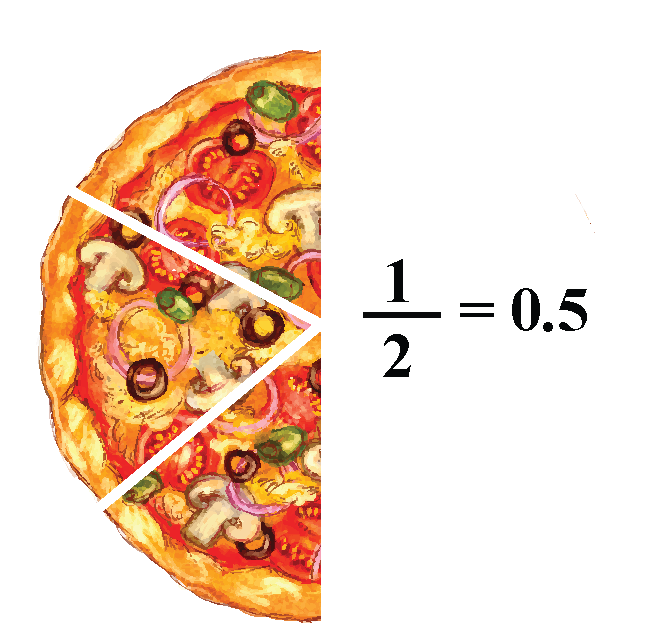Emma divides her garden into 12 equal parts. She grows flowers of different colors in each part of the garden.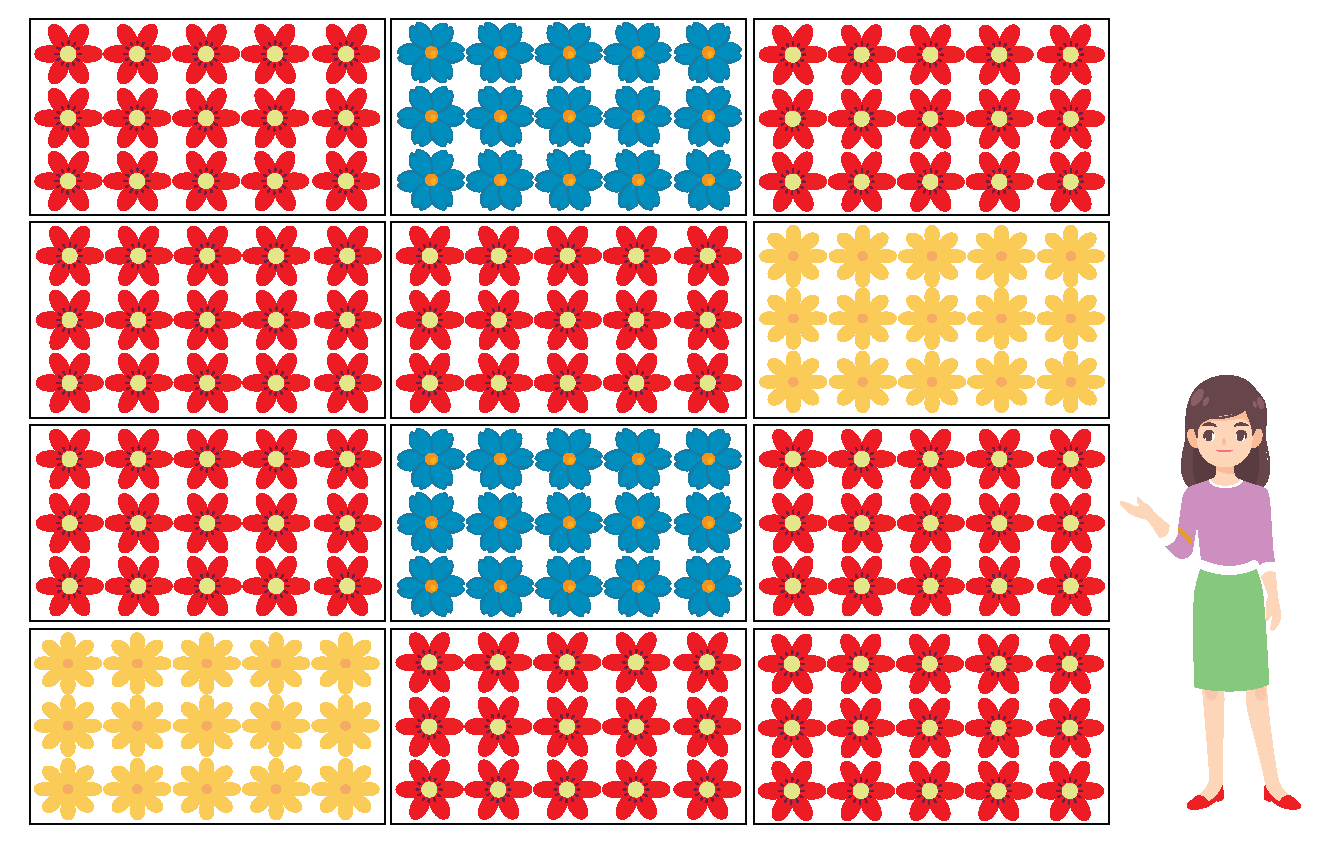Let us write the portion given to flowers of each color in fraction and in decimal.

Red flowers are grown in $$\dfrac{8}{12}$$ or $$0.666$$ part of the garden.

Yellow flowers are grown in $$\dfrac{2}{12}$$ or $$1.666$$ part of the garden.

Blue flowers are also grown in $$\dfrac{2}{12}$$ or $$1.666$$ part of the garden.

Let us look at the fraction and decimal chart to have more clarity about the relationship between fractions and decimals.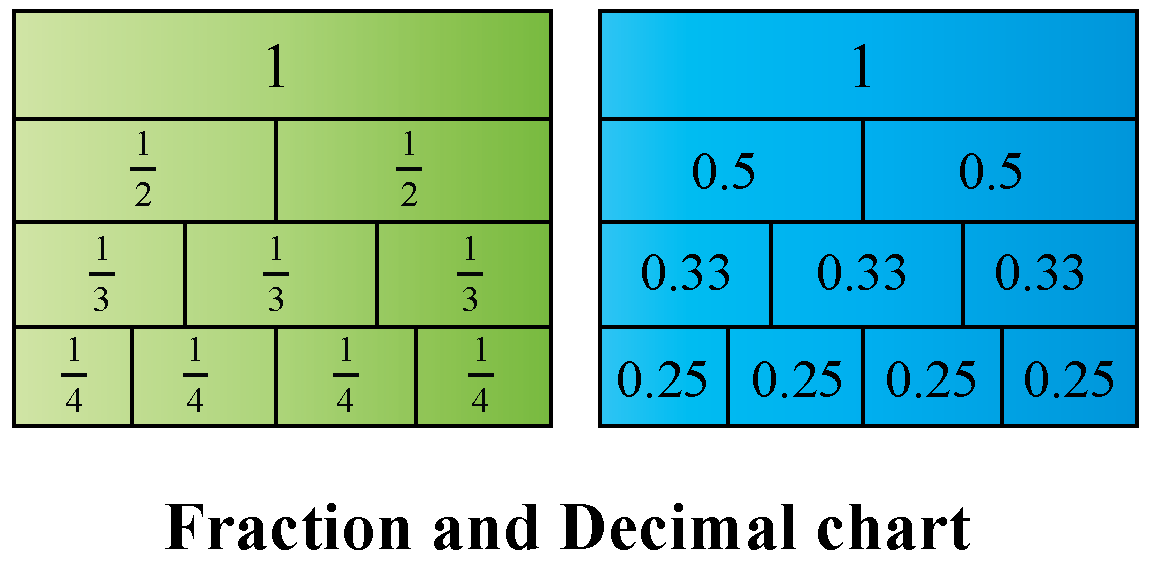Now, let us look at the fractional and decimal equivalents of some commonly used values.

 Fractional Value Decimal Value $$\dfrac{1}{2}$$ $$0.5$$ $$\dfrac{1}{3}$$ $$0.33$$ $$\dfrac{1}{4}$$ $$0.25$$ $$\dfrac{1}{6}$$ $$0.166$$ $$\dfrac{1}{7}$$ $$0.14$$ $$\dfrac{1}{8}$$ $$0.125$$ $$\dfrac{1}{9}$$ $$0.11$$ $$\dfrac{1}{10}$$ $$0.1$$ $$\dfrac{1}{20}$$ $$0.05$$ $$\dfrac{1}{25}$$ $$0.04$$ $$\dfrac{1}{50}$$ $$0.02$$

## How to Convert Fraction into Decimal?

We can convert a fraction to its decimal form by the following two methods.

• Long Division Method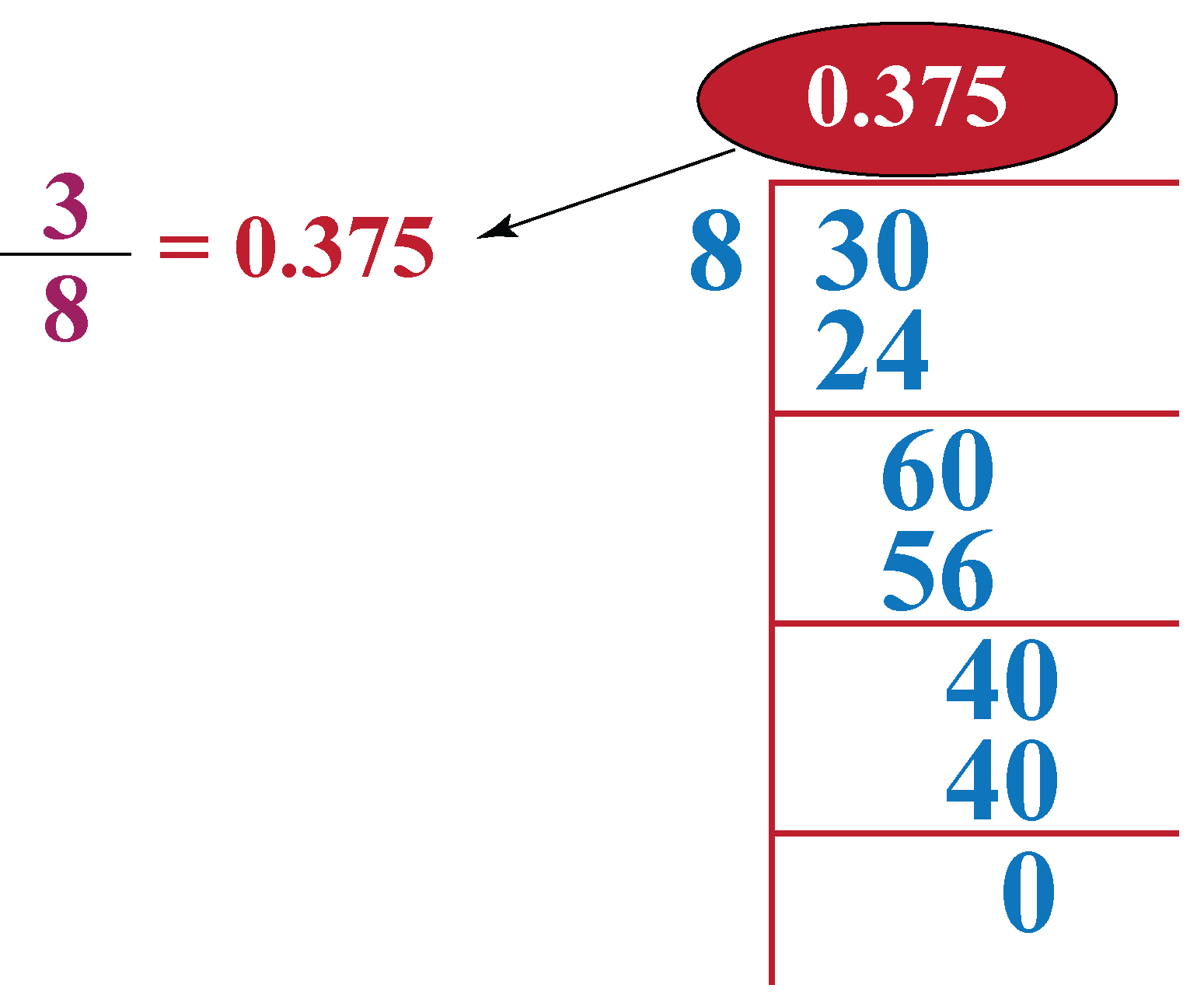• Convert the denominator of the fraction to multiples of 10 like 10, 100, 1000, etc.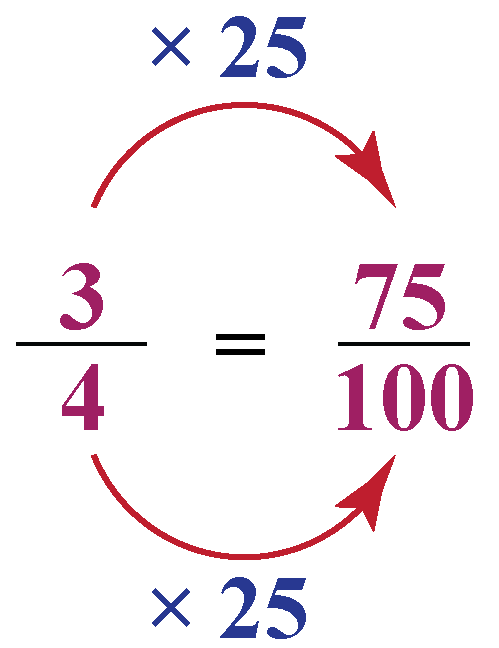### Fraction to Decimal Calculator

In the simulation given below, write any fraction and click on "Convert" to find its decimal equivalent.

## How to Convert Decimal into Fraction?

Every decimal number can be expressed in the form of a fraction. Steps to convert a decimal number to the fractional form are stated below:

1. Rewrite the number by ignoring the decimal point.
2. Divide the number by the place value of the last digit in the fractional part of the number.
3. Simplify the fraction.

Look at this example for a deeper understanding.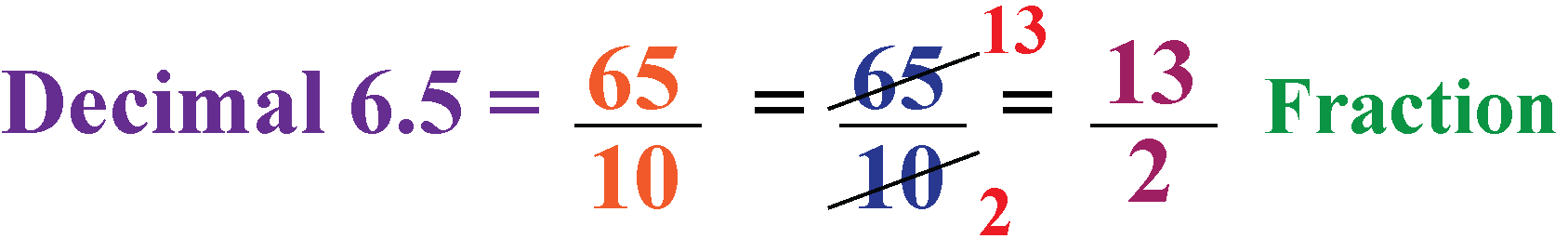### Decimal to Fraction Calculator

Decimal to fraction conversion calculator makes calculations easy.

In the simulation given below, write any decimal number and click on "Convert" to find the fraction equivalent to it.

## 4. How to convert decimals into fractions?

To convert a decimal to fraction, we follow three basic steps mentioned below:

• Rewrite the number by ignoring the decimal point
• Divide the number by the place value of the last digit in the fractional part of the number
• Simplify the fraction

## 5. What is 22/7 in decimals?

$$\dfrac{22}{7}$$ is an irrational number, which is expressed as $$3.14$$ in decimals up to two decimal places.

## 6. What are the ways to convert fraction to decimal?

There are two ways to convert a fraction into a decimal, which are given below:

• Long division method
• Conversion of denominator to powers of 10

## 7. How to convert a mixed fraction to decimal?

To convert a mixed fraction to decimal, we first need to convert it into an improper fraction. Then we can divide the numerator by denominator to convert it into decimal.

More Important Topics
Numbers
Algebra
Geometry
Measurement
Money
Data
Trigonometry
Calculus